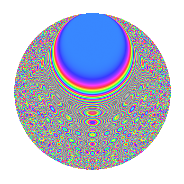# Properties

 Label 731.2.kLevel 731 Weight 2 Character orbit k Rep. character $$\chi_{731}(35,\cdot)$$ Character field $$\Q(\zeta_{7})$$ Dimension 360 Newforms 2 Sturm bound 132 Trace bound 2

# Related objects

## Defining parameters

 Level: $$N$$ = $$731 = 17 \cdot 43$$ Weight: $$k$$ = $$2$$ Character orbit: $$[\chi]$$ = 731.k (of order $$7$$ and degree $$6$$) Character conductor: $$\operatorname{cond}(\chi)$$ = $$43$$ Character field: $$\Q(\zeta_{7})$$ Newforms: $$2$$ Sturm bound: $$132$$ Trace bound: $$2$$ Distinguishing $$T_p$$: $$2$$

## Dimensions

The following table gives the dimensions of various subspaces of $$M_{2}(731, [\chi])$$.

Total New Old
Modular forms 408 360 48
Cusp forms 384 360 24
Eisenstein series 24 0 24

## Trace form

 $$360q - 4q^{2} - 4q^{3} - 68q^{4} - 4q^{5} - 8q^{6} + 12q^{7} + 16q^{8} - 44q^{9} + O(q^{10})$$ $$360q - 4q^{2} - 4q^{3} - 68q^{4} - 4q^{5} - 8q^{6} + 12q^{7} + 16q^{8} - 44q^{9} - 16q^{10} - 8q^{11} - 28q^{12} - 10q^{13} + 16q^{14} + 10q^{15} - 116q^{16} - 48q^{18} - 24q^{19} - 40q^{20} - 56q^{21} - 44q^{22} + 4q^{23} - 52q^{24} - 52q^{25} + 20q^{26} - 16q^{27} - 68q^{28} - 32q^{29} - 26q^{30} + 72q^{31} + 50q^{32} - 34q^{33} - 4q^{34} - 8q^{35} + 416q^{36} - 20q^{37} + 64q^{38} + 24q^{39} + 24q^{40} - 6q^{41} - 60q^{42} - 20q^{43} + 148q^{44} + 4q^{45} - 58q^{46} - 14q^{47} + 24q^{48} + 340q^{49} - 52q^{50} - 8q^{51} + 32q^{52} + 16q^{53} - 84q^{54} + 46q^{55} - 96q^{56} + 80q^{57} + 38q^{58} + 22q^{59} + 8q^{60} - 74q^{61} - 16q^{62} - 66q^{63} - 36q^{64} - 8q^{65} + 4q^{66} - 32q^{67} + 52q^{69} + 8q^{70} - 62q^{71} - 66q^{72} - 48q^{73} - 70q^{74} - 66q^{75} - 104q^{76} + 8q^{77} - 96q^{78} - 108q^{79} + 268q^{80} - 80q^{82} + 8q^{83} + 20q^{85} + 96q^{86} - 12q^{87} - 136q^{88} - 26q^{89} + 154q^{90} - 80q^{91} - 144q^{92} - 124q^{93} - 16q^{94} + 68q^{95} - 96q^{96} - 6q^{97} - 48q^{98} - 112q^{99} + O(q^{100})$$

## Decomposition of $$S_{2}^{\mathrm{new}}(731, [\chi])$$ into irreducible Hecke orbits

Label Dim. $$A$$ Field CM Traces $q$-expansion
$$a_2$$ $$a_3$$ $$a_5$$ $$a_7$$
731.2.k.a $$180$$ $$5.837$$ None $$-4$$ $$-6$$ $$-6$$ $$66$$
731.2.k.b $$180$$ $$5.837$$ None $$0$$ $$2$$ $$2$$ $$-54$$

## Decomposition of $$S_{2}^{\mathrm{old}}(731, [\chi])$$ into lower level spaces

$$S_{2}^{\mathrm{old}}(731, [\chi]) \cong$$ $$S_{2}^{\mathrm{new}}(43, [\chi])$$$$^{\oplus 2}$$